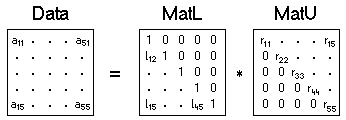The SDL Component Suite is an industry leading collection of components supporting scientific and engineering computing. Please visit the SDL Web site for more information....## LUDecomposition

 Unit: SDL_matrix Class: Declaration: function LUdecomposition (var MatL, MatU: TMatrix): boolean;

The method LUDecomposition decomposes the matrix into two triangular matrices MatL and MatU, with the matrix MatL being the lower triangular matrix (containing all 1s in the diagonal), and matrix MatU being the upper triangular matrix. The figure below shows the LU decomposition of a matrix of order 5.The function returns TRUE if the LU decomposition has been calculated successfully. Note that the product of the diagonal elements of matrix MatU is equal to the determinant of the matrix.

Last Update: 2016-Okt-02